Previous in Forum: GE MK6 Next in Forum: Advantech WebAccess Timer Script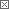Active Contributor

Join Date: Nov 2015
Posts: 14

#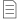Fuel Gas Volume Flow Compensation Formula

04/02/2017 9:49 AM

As known , To convert from uncompensated volume gas flow to compensated volume gas flow through orifice , the used equation is :
Fcomp = Funcomp X √((Pop/Pd)X (Td/Top)X (Gd/Gop)
Where G is the density , d:design (used in orifice sizing sheet) & Op. : operating
But sometimes it is mentioned in some text books with MW (Molecular weight) ?? Is it same ?? . Can anyone please give me details how we got this equation as I found it in some text books reversed i.e. (MWop / MWd) ?

Pathfinder Tags: Flow compensation gas
Interested in this discussion?
You can "subscribe" to this discussion to be notified of new comments.
Click on the Subscribe menu at the top of the page.

Check out these comments that don't yet have enough votes to be "official" good answers and, if you agree with them, rate them!
Guru

Join Date: Sep 2016
Posts: 918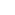#1

###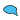Re: Fuel Gas Volume Flow Compensation Formula

04/02/2017 12:42 PM

It's just mass balance of a gas and difference of mass between in and out must be equal to zero.

Consider ideal gas equation PV=mRT, m=PV/RT, ρ=m/V=P/RT

Since, T_out≠T_in, P_out≠P_in then you would have a compensation factor for volume at in and out ports.

What's with the MW? MW does not change on the stream. MW is acquainted with R specific gas constant and cancelled out eventually.

Even so, I think out of convenience and clairvoyance most of the times. I might be wrong.DO READ ITS MANUAL, for good sake. Will you?

Guru

Join Date: Jul 2005
Location: Stoke-on-Trent, UK
Posts: 4281#2

###Re: Fuel Gas Volume Flow Compensation Formula

04/02/2017 1:47 PM

There's something wrong there IMHO. Firstly there's a close bracket missing from the end of the expression.

You don't need the (Gd/Gop). Gd/Gop = (Pd/Pop)X (Top/Td), so it cancels and you're left with Fcomp = Funcom.

If it's calibrated for gas of MWd, but used on gas of MWop, you would multiply by √(MWd/MWop), giving Fcomp = Funcomp X √((Pop/Pd)X (Td/Top)X (MWd/MWop)).

__________________
Give masochists a fair crack of the whip
Active Contributor

Join Date: Nov 2015
Posts: 14#3

###Re: Fuel Gas Volume Flow Compensation Formula

04/03/2017 3:39 AM

You are correct Codemaster, there is typo mistake, missing close bracket from the end.

Can you please explain how you get this equation:

Fcomp = Funcomp X √((Pop/Pd)X (Td/Top)X (MWd/MWop)) ????

I need to know the derivation/source of this equation.

Guru

Join Date: Jul 2005
Location: Stoke-on-Trent, UK
Posts: 4281#4

###Re: Fuel Gas Volume Flow Compensation Formula

04/03/2017 5:37 AM

OK

I assume you're now happy with Fcomp = Funcomp X √((Pop/Pd)X (Td/Top)) for case when the actual gas is same as design (calibration) gas.

If the actual gas is different, need also to correct for molecular weight. Eg if design gas is air, MW 29, but actual gas is hydrogen, MW 2, for a given flow the meter ΔP is lower, due to lower density, and correction factor is √(MWd/MWop), (> 1). The √ because ΔP varies as Q2.

Come back if you need more.

__________________
Give masochists a fair crack of the whip
Active Contributor

Join Date: Nov 2015
Posts: 14#5

###Re: Fuel Gas Volume Flow Compensation Formula

04/03/2017 11:30 AM

Thanks Code master , Please I tried to get this equation source as you can see in the attached file : http://s000.tinyupload.com/?file_id=86416981636594689620

But unable to get this equation, can you please advise what is wrong with my trial??

Guru

Join Date: Jul 2005
Location: Stoke-on-Trent, UK
Posts: 4281#6

###Re: Fuel Gas Volume Flow Compensation Formula

04/03/2017 1:14 PM

That link needs me to download software and is blocked by my security. Can you send the file in another form, or paste text directly into CR4?

__________________
Give masochists a fair crack of the whip
Active Contributor

Join Date: Nov 2015
Posts: 14#7

###Re: Fuel Gas Volume Flow Compensation Formula

04/04/2017 10:03 AM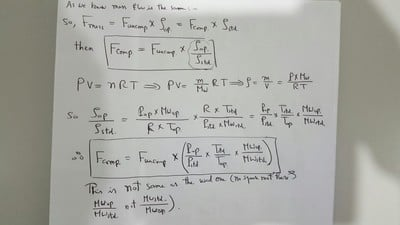Guru

Join Date: Jul 2005
Location: Stoke-on-Trent, UK
Posts: 4281#8

###Re: Fuel Gas Volume Flow Compensation Formula

04/04/2017 11:47 AM

Thanks.

You can't just convert the gas flows in inverse proportion to densities. If your orifice meter gives a certain ΔP at a certain mass flow and density, and then you double the gas density (eg double the pressure at constant temperature) keeping mass flow constant, as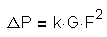the ΔP is halved (F2 by 1/4, G by 2)

For a given ΔP, which is what is being considered here,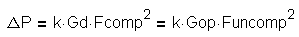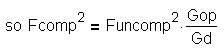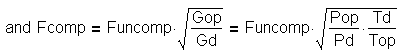Trust that helps.

__________________
Give masochists a fair crack of the whip
Active Contributor

Join Date: Nov 2015
Posts: 14#9

###Re: Fuel Gas Volume Flow Compensation Formula

04/04/2017 1:00 PM

Thanks Codematser,

I see you substitute G by P X T under the square root. According to ideal gas law, PV=nRT so G = m/V = P X MW / (RXTXZ); if we replace Gop / Gd from this equation then we will get Gop/ Gd = (Pop/Pd) X(Td/Top) X (MWop / MWd). My concern here is mainly for molecular weight correction it will be according to this substitution Sqrt(MWop/MWd); however, you mentioned in your reply #2 that the MW correction is Sqrt(MWd/MWop). Why did this contradiction happen?? From where do you get the MW correction to be Sqrt(MWd/MWop) ??

Guru

Join Date: Jul 2005
Location: Stoke-on-Trent, UK
Posts: 4281#10

###Re: Fuel Gas Volume Flow Compensation Formula

04/04/2017 3:10 PM

Let’s say the meter is calibrated for gas of mol wt MWd, and this gives ΔP1 at a given volume flow F, which is indicated on the scale.

If then the gas changes to MWop, volume flow unchanged, gas density changes in the same ratio, and ΔP changes to ΔP1*MWop/MWd (being proportional to density).

The indicated flow changes to F*√(MWop/MWd) (as indicated flow varies as √ΔP). So to get back to the correct volume flow F, need to multiply the reading by √(MWd/MWop)

__________________
Give masochists a fair crack of the whip
Active Contributor

Join Date: Nov 2015
Posts: 14#12

###Re: Fuel Gas Volume Flow Compensation Formula

04/05/2017 12:49 PM

I agree with you until (ΔP2= ΔP1*MWop/MWd) to keep same volume flow unchanged.

From this we can say : Find/Fd = Sqrt ((ΔP2/ ΔP1) X (MWd/Mwop)) ( Eqn.1)

(that’s why you said to keep Find = Fd and if Mwop increased from MWd then ΔP2 should increase from ΔP1 by same ratio) so till here we agreed completely.

But from same Eqn.1 , for same ΔP (i.e. for ΔP2 = ΔP1) , Find = Fd Sqrt (MWd/Mwop) so to correct Find to Fd, we have to multiply Find by : Sqrt (MWop/Mwd) not Sqrt (MWd/Mwop), this is where I am confused.

Guru

Join Date: Jul 2005
Location: Stoke-on-Trent, UK
Posts: 4281#13

###Re: Fuel Gas Volume Flow Compensation Formula

04/05/2017 1:29 PM

If MWd > MWop (eg d is air, op is hydrogen) for a given volume flow ΔP is lower on op, leading to lower indicated flow. So to bring indicated flow back to the correct figure, need to multiply by factor > 1. MWd/MWop > 1, MWop/MWd < 1. As I said in #4.

__________________
Give masochists a fair crack of the whip
Guru

Join Date: Jul 2005
Location: Stoke-on-Trent, UK
Posts: 4281#11

###Re: Fuel Gas Volume Flow Compensation Formula

04/05/2017 12:13 PM

A couple more thoughts on this, if it helps.

Don’t confuse 2 different scenarios.

If you have a known actual flow Fa at known Pa and Ta, you can convert this to other P and T conditions using P*V/T = constant, so for Pd and Td, Fd = Fa*(Pa/Pd)*(Td/Ta). This what’s going on (I think) in your attachment.

But that’s not the same as converting a flow reading on an orifice-plate flowmeter to other conditions (or other gas of different mol wt).

You can set up the meter to read (say) standard (15°C, 1 atm) flow at specified actual P and T. If you don’t need great accuracy and P and T aren’t going to vary much, you may not need to correct for actual P and T. Eg if P varies 5 – 6 bara, and T varies 90 – 100°C you could calibrate to read standard flow when actual P = 5.5 bara and T = 95°C.

Note this only applies to ΔP instruments with ΔP = k*F2 . It’s different for eg a rotating-piston type (typical domestic gas meter) or a vortex-shedding type.

__________________
Give masochists a fair crack of the whip
Participant

Join Date: Jul 2017
Posts: 2#14

###Re: Fuel Gas Volume Flow Compensation Formula

07/01/2017 9:37 AM

I'm having the exact same problem. I don't understand why the design and actual MWs are inverted on the standard flow compensation. Everything appears to work out fine for volumetric and mass flow compensation. Consider the following:

P/ρ = Z*(1545/MW)*T or ρ ∝ (P*MW)/(Z*T)

Qmeas = K * (√ΔP/√ρdes) and mmeas = K * (√ΔP*√ρdes)

meas = measured, des = design conditions, act = actual conditions

Volumetric Flow Compensation

Qact = K * (√ΔP/√ρdes) * (√ρdes/√ρact), or

Qact = Qmeas * √(Pdes/Pact) * √(MWdes/MWact)*√(Zact/Zdes)*√(Tact/Tdes)

Mass Flow Compensation

mact = K * (√ΔP * √ρdes) * (√ρact/√ρdes), or

mact = mmeas * √(Pact/Pdes) * √(MWact/MWdes)*√(Zdes/Zact)*√(Tdes/Tact)

Standard Flow Compensation

Qsmeas = Qmeas * (ρdesstd) = K * (√ΔP/√ρdes)*(ρdesstd) =K* (√ΔP * √ρdes)/ρstd, or

Qsact = K* (√ΔP * √ρdes)/ρstd * (√ρact/√ρdes) = Qsmeas * (√ρact/√ρdes), or

Qsact = Qsmeas * √(Pact/Pdes) * √(MWact/MWdes)*√(Zdes/Zact)*√(Tdes/Tact)

But the formula for standard flow compensation uses √(MWdes/MWact) - why?

Guru

Join Date: Jul 2005
Location: Stoke-on-Trent, UK
Posts: 4281#15

###Re: Fuel Gas Volume Flow Compensation Formula

07/01/2017 1:06 PM

I haven't digested all of that, but a couple of comments.

I assume your Z is compressibility factor. Maybe that needs to be taken account of in high accuracy work, but for the basic queries OP was making I think we can assume all gases considered are ideal so Z = 1.

In Qmeas = K * (√ΔP/√ρdes) and mmeas = K * (√ΔP*√ρdes) I think your confusing meas and des. For a given meter on a given gas, Q = K*√ΔP/√ρ = K*√(ΔP/ρ) for any Q and ρ, provided they are both measured at the same temp and pressure.

K can be calculated from first principles, using orifice dia. etc, and then if you just measure ΔP and you know ρact, (which you get from P, T and MW), you can calculate Qact. But the meter would normally be set up to read flow at some standard conditions (typically 15°C, 1 atm) at predetermined design density ρdes. If the actual density differs from ρdes, reading is multiplied by √(ρactdes).

If it's a gas with different MW, I gave my thinking in #10 and #13, I still that is correct.

__________________
Give masochists a fair crack of the whip
Guru

Join Date: Jul 2005
Location: Stoke-on-Trent, UK
Posts: 4281#16

###Re: Fuel Gas Volume Flow Compensation Formula

07/04/2017 12:38 PM

Just to recap

I think we need to consider 3 flow conditions

Standard conditions, Qstd, ρstd, the units the flow reading is usually wanted in, typically 15°C, 1 atm in US.

Actual conditions, Qact, ρact, of the flowing gas.

Calibration conditions, Qcal, ρcal, the Q and ρ at which the meter is set up to read standard flow.

I think those subscripts make it clearer than those used earlier.

When just working out relationships between various flow rates. It’s best to just use ρ, it keeps formulas simpler, and ρ can be put in terms of P and T at the end.

As I said before, if you’re working from first principles, you measure ΔP, calculate Qact = K*√(ΔP/ρact) and Qstd = Qactactstd.

If you’re starting from scratch, you might use a ΔP indicator with circular scale, deflection linear with ΔP. You have Qcal = K*√(ΔP/ρcal) and Qstd = Qcalcalstd = K*√(ΔP*ρcal)/ρstd.

You then mark on the scale Qstd at a range of ΔP. Of course the flow readings are not linear with deflection. As long as ρact is same as ρcal it’s fine, if it’s not, determined by P and T instruments, the reading is corrected by multiplying by √(ρactcal).

If it’s a more sophisticated set-up, with instruments giving outputs to a PLC, it would be set up to do the corrections for you.

If it’s then used on a gas of different MW, need to use that to find ρcal. Also in the PLC case need to reconfigure it, as it will not know from the P and T inputs.

__________________
Give masochists a fair crack of the whip
Participant

Join Date: Jul 2017
Posts: 2#17

###Re: Fuel Gas Volume Flow Compensation Formula

07/06/2017 12:48 PM

Guru

Join Date: Jul 2005
Location: Stoke-on-Trent, UK
Posts: 4281#18

###Re: Fuel Gas Volume Flow Compensation Formula

07/06/2017 1:22 PM

OK, it takes a bit of getting one's head round (mine included). OP's nomenclature was harder to follow as he also hadn't bothered to use subscripts.

Come back if you can find any errors or want to discuss further.

__________________
Give masochists a fair crack of the whip
Participant

Join Date: Nov 2015
Posts: 1#19

###Re: Fuel Gas Volume Flow Compensation Formula

08/22/2017 12:57 AM

Codemaster,

I am confused about how you derived this equation:

ΔP = k.GD.Fcomp2 = k.Gop.Funcomp2

Taking a step back, we know that

F= k √(ΔP/G)

I was of the view that the uncompensated flow is based on the design/calibration density, i.e.

Funcomp = k √(ΔP/GD)

The goal for us is to then convert this uncompensated flow to a compensated flow based on actual operating conditions.

ΔP = k.GD.Funcomp2 = k.Gp.Fcomp2

However, this would result in:

Fcomp = Funcomp .√((PD/Pop).(Top/TD))

Which does not align with your final formula.

I am not doubting that your final compensation equation is correct, but I would like to better understand how it has been derived.

Thanks for your help so far.

Guru

Join Date: Jul 2005
Location: Stoke-on-Trent, UK
Posts: 4281#20

###Re: Fuel Gas Volume Flow Compensation Formula

08/22/2017 1:17 PM

pleco4me

In ΔP = k.GD.Fcomp2 = k.Gop.Funcomp2, GD and Fcomp are both at design conditions, Gop and Funcomp are both at operating conditions. As I said in #15, for a given meter on a given gas, Q = K*√ΔP/√ρ = K*√(ΔP/ρ) for any Q and ρ, provided they are both measured at the same temp and pressure. So ΔP = K*ρ*Q2, provided ρ and Q are at same conditions.

So your Funcomp = k √(ΔP/GD) is not correct, it should be Funcomp = k √(ΔP/Gop) and Fcomp = k √(ΔP/GD), giving Fcomp = Funcomp*√(Gop/GD).

I would like to use the scheme I suggested in #16, as I think we're getting a bit confused with nomenclature. It's better IMO to use the same subscript for standard (std) for what you call D and comp, and actual (act) for your op and uncomp. Forget about calibration conditions if you prefer. It only comes into it if you've set up the meter to read std flow at one set of actual conditions (which would be the calibration conditions) but the actual actual conditions (!) are different.

Also I had another look at hanywepco's #7. It's OK if the gas density changes due to a change in P and T, but if the volume flowrate (actual or standard) stays constant but the MW changes, the ΔP changes proportional to the MW. That has the effect of inverting MWd/Mwop. I explained it in #10 and #13. If you think of a situation where the only change is MW, eg design is air, operating is hydrogen, you can see the flow reading has to be adjusted by a factor > 1.

Trust that helps!

__________________
Give masochists a fair crack of the whip
Participant

Join Date: Jan 2021
Posts: 1#21

###Re: Fuel Gas Volume Flow Compensation Formula

01/31/2021 10:20 AM

Hi,

First, I know this is old, but it still comes up on a Google search. So hopefully my answer will help someone in the future.

Let's look at some equations. Starting with mass flow, we will build up to standard volumetric flow after. The mass flow through an orifice plate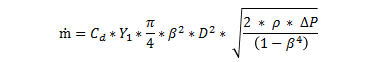m = FMC * √(ΔP)

FMC is thus everything except for the ΔP reading. Note the FMC includes the density at design conditions (which we will be compensating for). So when a ΔP signal is read, square root extracting is done, and then multiplied by a constant (FMC) to get the flow in Sm3/hr (or MMSCFD).

Let's take the density back out of the FMC.

m = FMC' * √(ΔP) * √(ρdes)

Where FMC' = FMC / √(ρdes) (we have extracted it out... for now) and ρdes is the density that was used during the design calculations

The equation for Real Gases

ρ = (MW * P) / (Z * R * T)

I'm going to use R, the Gas Constant, as the only number that would be equal between two gasses. So to compare two gasses;

(MW1 * P1) / (Z1 * ρ1 * T1) = (MW2 * P2) / (Z2 * ρ2 * T2)

Assuming that MW and Z change little from original design conditions, we can cancel these out.

P1 / (ρ1 * T1) = P2 / (ρ2 * T2)

ρ1 = (ρ2 * P1 * T2) / (T1 * P2)

So

ρop = des * Pop * Tdes) / (Top * Pdes)

Back to our equation

m = FMC' * √(ΔP) * √(ρdes)

Substituting in what the density is now calculated to be (ρop)

m = FMC' * √(ΔP) * √[ (ρdes * Pop * Tdes) / (Top * Pdes) ]

m = [ FMC' * √(ρdes) ] * √(ΔP) * √[ (Pop / Pdes) * (Tdes / Top) ]

m = FMC * √(ΔP) * √[ (Pop / Pdes) * (Tdes / Top) ]

Our FMC can remain the same, and we have compensated the reading.

What if our gas changes so dramatically, that we can no longer assume MW and Z are the similar? We would want to take a gas sample, and update the density. Extracting out ρdes from the FMC again

m = [ FMC' * √(ρdes) ] * √(ΔP)

Let's multiply it by √(ρop) / √(ρdes). This would cancel out √(ρdes) from the FMC.

m = [ FMC' * √(ρdes) ] * √(ΔP) * √(ρop) / √(ρdes)

m = FMC * √(ΔP) * √(ρop / ρdes)

So we haven't had to touch the original FMC (or range on the DCS). What if we now want to use P/T compensation.

ρop_new = op * Pop_new * Top) / (Top_new * Pop)

Substituting in what the density is now calculated to be (ρop_new)

m = FMC * √(ΔP) * √[ (ρop * Pop_new * Top) / (Top_new * Pop) / ρdes ]

m = FMC * √(ΔP) * √[ (Pop_new / Pop) * (Top / Top_new) * (ρop / ρdes) ]

Notice that your original (ρop / ρdes) is still there after rearranging? So based on this, you could periodically take a gas sample, and calculate the new ρop (noting Top and Pop) and update those 3 parameters in your calculation. ρdes would remain the same (as it exists in the original FMC and needs to be cancelled out). And you just need to measure T and P.

Let's look at how MW can be used. This is important for when we look at standard volumetric flow. This time, we will assume that MW can change, however, we will still consider the change in Z to be negligible.

ρ1 = (ρ2 * P1 * T2 * MW1) / (T1 * P2 * MW2)

So

ρop = des * Pop * Tdes * MWop) / (Top * Pdes * MWdes)

Back to our equation

m = FMC' * √(ΔP) * √(ρdes)

Substituting in what the density is now calculated to be (ρop)

m = FMC' * √(ΔP) * √[ (ρdes * Pop * Tdes * MWop) / (Top * Pdes * MWdes) ]

m = [ FMC' * √(ρdes) ] * √(ΔP) * √[ (Pop / Pdes) * (Tdes / Top) * (MWop / MWdes) ]

m = FMC * √(ΔP) * √[ (Pop / Pdes) * (Tdes / Top) * (MWop / MWdes)]

We've dealt with mass flow, but let's make things even more complicated. Let's look at standard volumetric flow (I use Q, whereas you've used F).

Q = m / ρstd

Q = [ FMC' * √(ΔP) * √(ρdes) ] / ρstd

So the use of FMC is the same. FMC now just incorporates ρstd

Q = FMCQ * √(ΔP)

We can use all the same equations as the above, leaving ρstd (which is incorporated inside FMCQ at the moment) uncompensated.

Q = FMCQ * √(ΔP) * √[ (Pop / Pdes) * (Tdes / Top) * (MWop / MWdes)]

So how can we compensate for ρstd?

Q = [ FMCQ' / ρstd ] * √(ΔP)

Let's consider this equation again

(MW1 * P1) / (Z1 * ρ1 * T1) = (MW2 * P2) / (Z2 * ρ2 * T2)

For standard density, P1=P2 (1 bar), T1=T2 (60 degF) and we can assume that Z1=Z2 (usually ~1). That leaves us with

MW1 / ρ1= MW2 / ρ2

As ρstd was calculated with the MW at operating and design conditions

MWop / ρstd_op = MWdes / ρstd_des

ρstd_new = (MWop / MWdes) * ρstd_des

Taking our standard volumetric flow equation

Q = FMCQ' * √(ΔP) / ρstd

Q = FMCQ' * √(ΔP) / [ (MWop / MWdes) * ρstd_des ]

Q = [ FMCQ' / ρstd_des ] * √(ΔP) / (MWop / MWdes)

Q = FMCQ * √(ΔP) * (MWdes / MWop)

Inserting the compensation we used in the mass flow calculation to compensate for ρop

Q = [ FMCQ * √(ΔP) * √[ (Pop / Pdes) * (Tdes / Top) * (MWop / MWdes) ] * (MWdes / MWop)

See what happens here? We have (MWop / MWdes) in a square root, and (MWdes / MWop) outside the square root. So it becomes

Q = FMCQ * [√(ΔP) * √[ (Pop / Pdes) * (Tdes / Top) * (MWdes / MWop)]

That's why you see it 'inverted' when dealing with standard volumetric flow as opposed to mass flow. The inversion occurs because you are compensating ρdes and ρstd_des.

Now back to your original equation.

Fcomp = Funcomp * √[ (Pop / Pdes) * (Tdes / Top) * (Gdes / Gop)]

Assuming F is standard volumetric flow (like my Q above) and G is density, I cannot work out any possible way to make this equation hold true. Only MW would work here, as shown above.

Interested in this discussion?
You can "subscribe" to this discussion to be notified of new comments.
Click on the Subscribe menu at the top of the page.